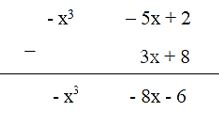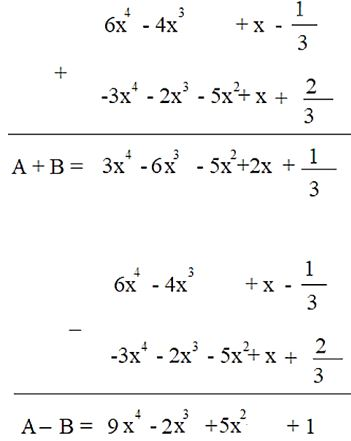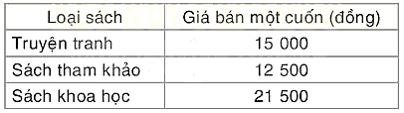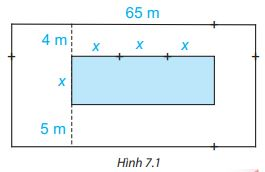## Solving Exercises Lesson 26 Addition and subtraction of one-variable polynomials (Chapter 7 Math 7 Connection) – Math Book

Solving Exercises Lesson 26 Addition and subtraction of one-variable polynomials (Chapter 7 Math 7 Connect)
============

### Solve problem 7.12, page 33, Math 7 textbook Connecting knowledge volume 2 – KNTT

Find the sum of the following two polynomials by grouping terms of the same degree: x2 – 3x + 2 and 4x3 – x2 + x – 1

## Detailed instructions for solving Problem 7.12

Solution method

Remove the brackets and group the terms of the same degree.

Detailed explanation

We have: (x2 – 3x + 2) + (4x .)3 – x2 + x – 1)

= x2 – 3x + 2 + 4x3 – x2 + x – 1

= 4x3 + (x2 – x2 ) + (-3x + x) + (2 – 1)

= 4x3 – 2x + 1

–>

— *****

### Solve problem 7.13 page 33 Math 7 Textbook Connecting knowledge volume 2 – KNTT

Find the following difference by setting subtraction: $$( – {\rm{ }}{x^3}–5x + 2)–\left( {3x + 8} \right)$$

## Detailed instructions for solving problem 7.13

Solution method

Set the subtraction so that the terms of the same degree are placed in the same column, and then subtract each column.

Detailed explanation–>

— *****

### Solve problem 7.14, page 33, Math 7 Textbook Connecting knowledge volume 2 – KNTT

Given two polynomials: $$A = 6{x^4} – 4{x^3} + x – \dfrac{1}{3};B = – 3{x^4} – 2{x^3} – 5{x^2} + x + \dfrac{2}{3}$$. Calculate A + B and A – B

## Detailed instructions for solving Problem 7.14

Solution method

Method 1: Remove the brackets and group the terms of the same degree.

Method 2: Set the addition (subtract) so that the terms of the same degree are placed in the same column and then add (subtract ) by each column.

Detailed explanation

Method 1:

$$\begin{array}{l}A + B = (6{x^4} – 4{x^3} + x – \dfrac{1}{3}) + ( – 3{x^4} – 2{x^3} – 5{x^2} + x + \dfrac{2}{3})\\ = 6{x^4} – 4{x^3} + x – \dfrac{1}{ 3} – 3{x^4} – 2{x^3} – 5{x^2} + x + \dfrac{2}{3}\\ = (6{x^4} – 3{x^4 }) + ( – 4{x^3} – 2{x^3}) – 5{x^2} + (x + x) + ( – \dfrac{1}{3} + \dfrac{2}{ 3})\\ = 3{x^4} – 6{x^3} – 5{x^2} + 2x + \dfrac{1}{3}\\A – B = (6{x^4} – 4{x^3} + x – \dfrac{1}{3}) – ( ​​– 3{x^4} – 2{x^3} – 5{x^2} + x + \dfrac{2} {3})\\ = 6{x^4} – 4{x^3} + x – \dfrac{1}{3} + 3{x^4} + 2{x^3} + 5{x^ 2} – x – \dfrac{2}{3}\\ = (6{x^4} + 3{x^4}) + ( – 4{x^3} + 2{x^3}) + 5 {x^2} + (x – x) + ( – \dfrac{1}{3} – \dfrac{2}{3})\\ = 9{x^4} – 2{x^3} + 5 {x^2} – 1\end{array}$$$$\begin{array}{l}A + B = (6{x^4} – 4{x^3} + x – \dfrac{1} {3}) + ( – 3{x^4} – 2{x^3} – 5{x^2} + x + \dfrac{2}{3})\\ = 6{x^4} – 4 {x^3} + x – \dfrac{1}{3} – 3{x^4} – 2{x^3} – 5{x^2} + x + \dfrac{2}{3}\\ = (6{x^4} – 3{x^4}) + ( – 4{x^3} – 2{x^3}) – 5{x^2} + (x + x) + ( – \ dfrac{1}{3} + \dfrac{2}{3})\\ = 3{x^4} – 6{x^3} – 5{x^2} + 2x + \dfrac{1}{3}\\A – B = (6{x^4} – 4{x^3} + x – \dfrac{1}{3}) – ( ​​– 3{x^ 4} – 2{x^3} – 5{x^2} + x + \dfrac{2}{3})\\ = 6{x^4} – 4{x^3} + x – \dfrac{ 1}{3} + 3{x^4} + 2{x^3} + 5{x^2} – x – \dfrac{2}{3}\\ = (6{x^4} + 3{ x^4}) + ( – 4{x^3} + 2{x^3}) + 5{x^2} + (x – x) + ( – \dfrac{1}{3} – \dfrac{ 2}{3})\\ = 9{x^4} – 2{x^3} + 5{x^2} – 1\end{array}$$

Method 2:–>

— *****

### Solve problem 7.15 page 33 Math textbook 7 Connecting knowledge volume 2 – KNTT

For polynomials: $$A = 3{x^4} – 2{x^3} – x + 1;B = – 2{x^3} + 4{x^2} + 5x;C = – 3 {x^4} + 2{x^2} + 5$$. Calculate A + B + C; A – B + C and A – B – C

## Detailed instructions for solving problem 7.15

Solution method

Remove the brackets and group the terms of the same degree.

Detailed explanation

$$\begin{array}{l}A + B + C = (3{x^4} – 2{x^3} – x + 1) + ( – 2{x^3} + 4{x^2 } + 5x) + ( – 3{x^4} + 2{x^2} + 5)\\ = 3{x^4} – 2{x^3} – x + 1 – 2{x^3} + 4{x^2} + 5x – 3{x^4} + 2{x^2} + 5\\ = (3{x^4} – 3{x^4}) + ( – 2{x^ 3} – 2{x^3}) + (4{x^2} + 2{x^2}) + ( – x + 5x) + (1 + 5)\\ = 0 + ( – 4{x^) 3}) + 6{x^2} + 4x + 6\\ = – 4{x^3} + 6{x^2} + 4x + 6\\A – B + C = (3{x^4} – 2{x^3} – x + 1) – ( ​​– 2{x^3} + 4{x^2} + 5x) + ( – 3{x^4} + 2{x^2} + 5) \\ = 3{x^4} – 2{x^3} – x + 1 + 2{x^3} – 4{x^2} – 5x – 3{x^4} + 2{x^2} + 5\\ = (3{x^4} – 3{x^4}) + ( – 2{x^3} + 2{x^3}) + ( – 4{x^2} + 2{x ^2}) + ( – x – 5x) + (1 + 5)\\ = 0 + 0 + ( – 2{x^2}) – 6x + 6\\ = – 2{x^2} – 6x + 6\\A – B – C = (3{x^4} – 2{x^3} – x + 1) – ( ​​– 2{x^3} + 4{x^2} + 5x) – ( ​​– 3{x^4} + 2{x^2} + 5)\\ = 3{x^4} – 2{x^3} – x + 1 + 2{x^3} – 4{x^2} – 5x + 3{x^4} – 2{x^2} – 5\\ = (3{x^4} + 3{x^4}) + ( – 2{x^3} + 2{x^ 3}) + ( – 4{x^2} – 2{x^2}) + ( – x – 5x) + (1 – 5)\\ = 6{x^4} + 0 + ( – 6{x ^2}) – 6x + ( – 4)\\ = 6{x^4} – 6{x^2} – 6x – 4\end{array}$$

–>

— *****

### Solve problem 7.16 page 33 Math 7 textbook Connecting knowledge volume 2 – KNTT

Nam’s friend was assigned to buy some books as gifts in the year-end review of the class. Nam plans to buy three kinds of books with the selling price as shown in the following table. Suppose Nam needs to buy x science books, x+8 reference books and x + 5 comic books.a) Write polynomials representing the amount Nam has to pay for each type of book.

b) Find the polynomial representing the total amount Nam has to pay to buy that number of books.

## Detailed instructions for solving Problem 7.16

Solution method

Write a polynomial representing the amount

Money to buy 1 type of book = number of books. price of a book

Detailed explanation

a) The polynomial representing the amount Nam has to pay for the comic is: A = (x +5). 15 000 = 15 000x + 75 000 (VND)

The polynomial representing the amount Nam has to pay for the reference book is: B = (x + 8) . 12 500 = 12 500x + 100 000 (VND)

The polynomial representing the amount Nam has to pay for science books is: C = x . 21 500 (VND)

b) The polynomial expressing the total amount Nam has to pay to buy that number of books is:

P = A + B + C = = 15 000x + 75 000 + 12 500x + 100 000 + x . 21 500

= (15 000 + 12 500 + 21 500)x + (75 000 + 100 000)

= 49 000x + 175 000 (VND)

–>

— *****

### Solve problem 7.17 page 33 Math textbook 7 Connecting knowledge volume 2 – KNTT

On a rectangular piece of land with a length of 65 m, a swimming pool is planned to have a width of x strokes, and the length is 3 times the width. Specific diagrams and dimensions (in meters) are shown in Figure 7.1. Find the polynomial (variable x):

a) Indicate the area of ​​the swimming pool

b) Indicate the area of ​​the land

c) Indicates the area of ​​land around the swimming pool.## Detailed instructions for solving Problem 7.17

Solution method

+ Indicates the length and width of the rectangles

Area of ​​rectangle = length. width

Detailed explanation

a) The pool has a length of 3x and a width of x, so the polynomial for the area of ​​the pool is:

B = 3x. x = 3.x2

b) The plot of land has a length of 65 and a width of 5 + x + 4 = x + 9, so the polynomial representing the area of ​​​​the land is:

D = 65. (x+9) = 65x + 585

c) The area around the swimming pool = the area of ​​​​the land – the area of ​​​​the swimming pool, so the polynomial expressing the area of ​​​​the land around the swimming pool is:

Q = D – B = 65x + 585 – 3.x2 = -3.x2 +65x + 585

–>

— *****